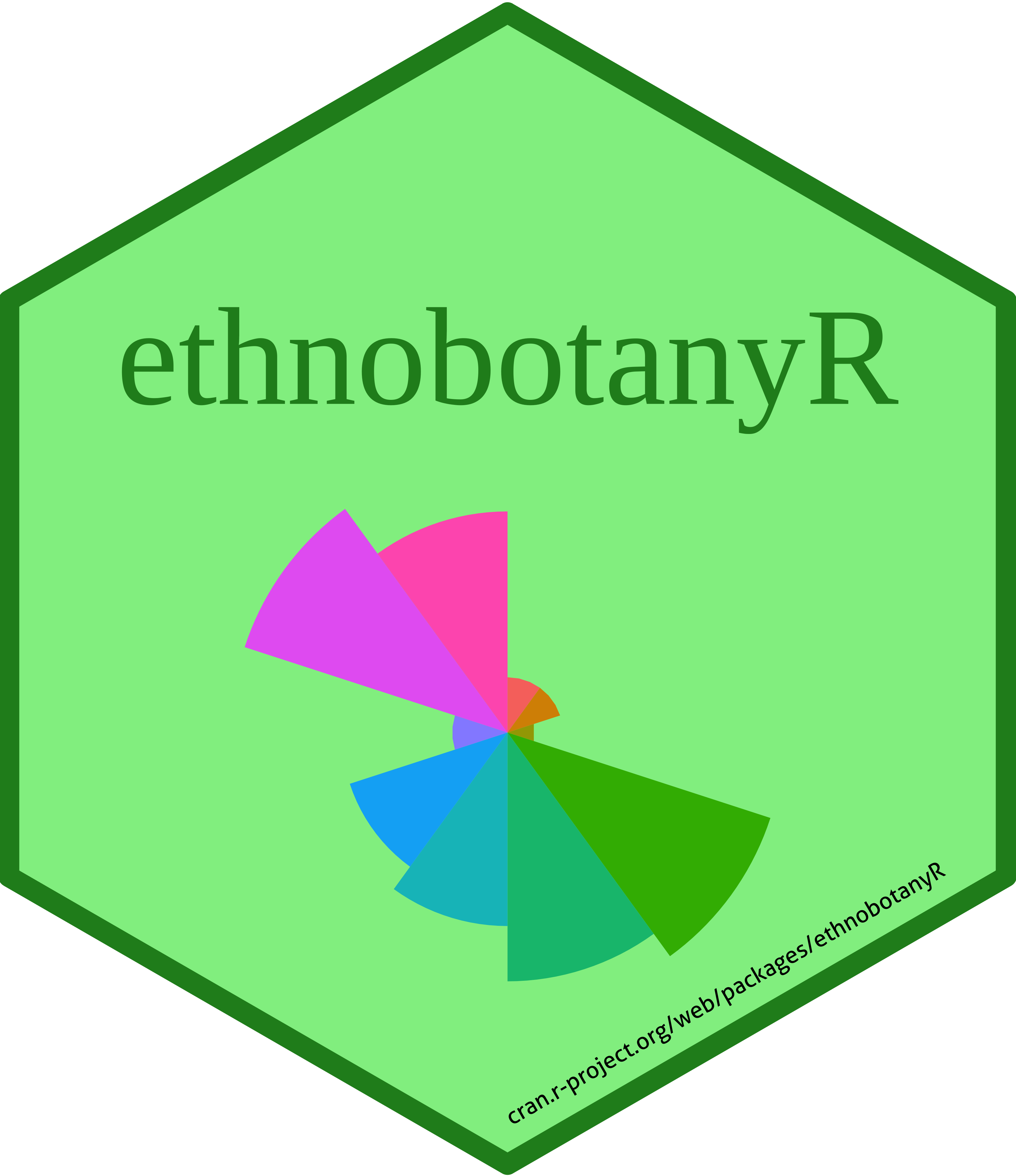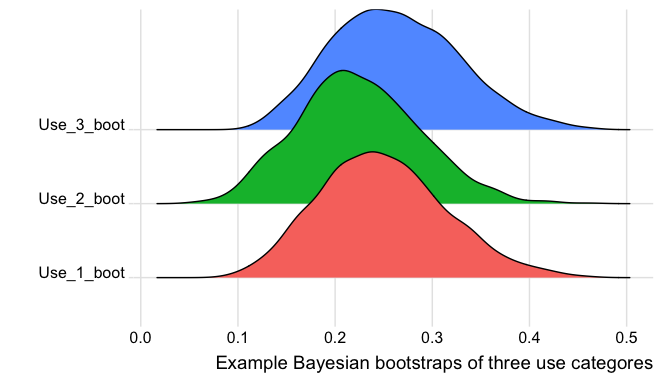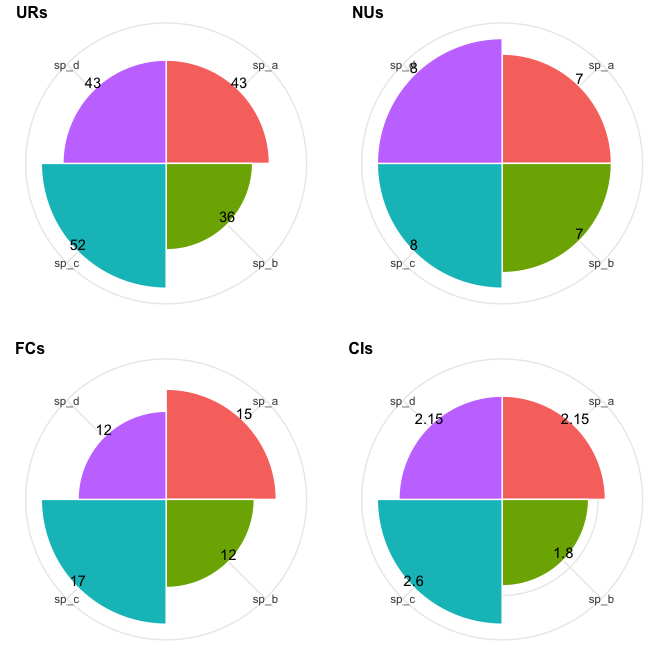# Quantitative ethnobotany analysis with ethnobotanyRThe ethnobotanyR package calculates common quantitative ethnobotany indices to assess the cultural significance of plant species based on informant consensus. The package closely follows two papers, one on cultural importance indices (Tardio and Pardo-de-Santayana 2008) and another on agrobiodiversity valuation (Whitney, Bahati, and Gebauer 2018). The goal is to provide an easy-to-use platform for ethnobotanists to calculate quantitative ethnobotany indices. Users are highly encouraged to familiarize themselves with ethnobotany theory (Gaoue et al. 2017; Albuquerque and Hurrell 2010) and social ecological theory (Albuquerque et al. 2019). An overview of this theoretical background will be helpful in understanding approaches in ethnobotany and formulating useful research questions.

An example data set called ethnobotanydata is provided to show how standard ethnobotany data should be formatted to interface with the ethnobotanyR package. This is an ethnobotany data set including one column of 20 knowledge holder identifiers informant and one of 4 species names sp_name. The rest of the columns are the identified ethnobotany use categories. The data in the use categories is populated with counts of uses per person (should be 0 or 1 values).1

Many of the functions in ethnobotanyR make use of select() and filter_all() functions of the dplyr package (Wickham et al. 2019) and pipe functions %>% from the magrittr package (Bache and Wickham 2014). These are easy to use and understand and allow users the chance to pull the code for these functions and change anything they see fit.

First six rows of the example ethnobotany data included with ethnobotanyR
informant sp_name Use_1 Use_2 Use_3 Use_4 Use_5 Use_6 Use_7 Use_8 Use_9 Use_10
inform_a sp_a 0 0 1 0 0 0 0 1 1 0
inform_a sp_b 0 0 0 0 0 0 0 0 0 0
inform_a sp_c 0 0 0 0 0 1 1 0 0 0
inform_a sp_d 0 0 0 0 0 0 0 0 0 0
inform_b sp_a 0 1 1 0 0 1 0 0 1 0
inform_b sp_b 1 0 0 0 0 0 1 0 0 0

## ethnobotanyR package functions

### Use Report (UR) per species

The use report URs() is the most basic ethnobotany calculation. The function calculates the use report (UR) for each species in the data set.

$\begin{equation} UR_{s} = \sum_{u=u_1}^{^uNC} \sum_{i=i_1}^{^iN} UR_{ui} \end{equation}$

URs() calculates the total uses for the species by all informants (from $$i_1$$ to $$^iN$$) within each use-category for that species $$(s)$$. It is a count of the number of informants who mention each use-category $$NC$$ for the species and the sum of all uses in each use-category (from $$u_1$$ to $$^uNC$$) (see Prance et al. 1987).

ethnobotanyR::URs(ethnobotanydata)
#>   sp_name URs
#> 1    sp_c  52
#> 2    sp_a  43
#> 3    sp_d  43
#> 4    sp_b  36

The URsum() function calculates the sum of all ethnobotany use reports (UR) for all species in the data set (see Prance et al. 1987).

ethnobotanyR::URsum(ethnobotanydata)
#>  174

### Cultural Importance (CI) index

The CIs() function calculates the cultural importance index (CI) for each species in the data set.

$\begin{equation} CI_{s} = \sum_{u=u_1}^{^uNC} \sum_{i=i_1}^{^iN} UR_{ui/N}. \end{equation}$

CIs() is essentially URs() divided by the number of informants to account for the diversity of uses for the species (see Tardio and Pardo-de-Santayana 2008).

ethnobotanyR::CIs(ethnobotanydata)
#>   sp_name   CI
#> 1    sp_c 2.60
#> 2    sp_a 2.15
#> 3    sp_d 2.15
#> 4    sp_b 1.80

### Frequency of Citation (FC) per species

The FCs() function calculates the frequency of citation (FC) for each species in the data set.

$\begin{equation} FC_s = \sum_{i=i_1}^{^iN}{UR_i} \end{equation}$

FCs() is the sum of informants that cite a use for the species (see Prance et al. 1987).

ethnobotanyR::FCs(ethnobotanydata)
#>   sp_name FCs
#> 1    sp_c  17
#> 2    sp_a  15
#> 3    sp_b  12
#> 4    sp_d  12

### Number of Uses (NU) per species

The NUs() function calculates the number of uses (NU) for each species in the data set.

$\begin{equation} NU_s = \sum_{u=u_1}^{^uNC} \end{equation}$

$$NC$$ are the number of use categories. NUs() is the sum of all categories for which a species is considered useful (see Prance et al. 1987).

ethnobotanyR::NUs(ethnobotanydata)
#>   sp_name NUs
#> 1    sp_c   8
#> 2    sp_d   8
#> 3    sp_a   7
#> 4    sp_b   7

### Relative Frequency of Citation (RFC) index

The RFCs() function calculates the relative frequency of citation (RFC) for each species in the data set.

$\begin{equation} RFC_s = \frac{FC_s}{N} = \frac{\sum_{i=i_1}^{^iN} UR_i}{N} \end{equation}$

$$FC_s$$ is the frequency of citation for each species $$s$$, $$UR_i$$ are the use reports for all informants $$i$$ and $$N$$ is the total number of informants interviewed in the survey (see Tardio and Pardo-de-Santayana 2008).

ethnobotanyR::RFCs(ethnobotanydata)
#>   sp_name RFCs
#> 1    sp_c 0.85
#> 2    sp_a 0.75
#> 3    sp_b 0.60
#> 4    sp_d 0.60

### Relative Importance (RI) index

The RIs() function calculates the relative importance index (RI) for each species in the data set.

$\begin{equation} RI_s = \frac{RFC_{s(max)}+RNU_{s(max)}}{2} \end{equation}$

$$RFC_{s(max)}$$ is the relative frequency of citation for the species $$s$$ over the maximum, $$RNU_{s(max)}$$ is the relative number of uses for $$s$$ over the maximum (see Tardio and Pardo-de-Santayana 2008).

ethnobotanyR::RIs(ethnobotanydata)
#>   sp_name   RIs
#> 1    sp_c 0.925
#> 2    sp_a 0.812
#> 3    sp_d 0.800
#> 4    sp_b 0.738

### Use Value (UV) index

The UVs() function calculates the use value (UV) index for each species in the data set.

$\begin{equation} UV_{s} = \sum_{i=i_1}^{^iN} \sum_{u=u_1}^{^uNC} UR_{ui/N} \end{equation}$

UVs() is essentially the same as CIs() except that it starts with the sum of UR groupings by informants. $$U_i$$ is the number of different uses mentioned by each informant $$i$$ and $$N$$ is the total number of informants interviewed in the survey (see Tardio and Pardo-de-Santayana 2008).

ethnobotanyR::UVs(ethnobotanydata)
#>   sp_name   UV
#> 1    sp_c 2.60
#> 2    sp_a 2.15
#> 3    sp_d 2.15
#> 4    sp_b 1.80

The simple_UVs() function calculates the simplified use value (UV) index for each species in the data set.

$\begin{equation} UV_{s} = \sum U_i/N \end{equation}$

$$U_i$$ is the number of different uses mentioned by each informant $$i$$ and $$N$$ is the total number of informants interviewed in the survey (see Albuquerque et al. 2006).

### Cultural Value (CVe) for ethnospecies

The CVe() function calculates the cultural value (CVe) for ethnospecies. The index is one of three proposed for assessing the cultural, practical and economic dimensions (ethno) species importance. Reyes-Garcia et al. (2006) suggest several more indices but $$CV_e$$ is the most commonly used from that study (Reyes-Garcia et al. 2006).

$\begin{equation} CV_{e} = {Uc_{e}} \cdot{IC_{e}} \cdot \sum {IUc_{e}} \end{equation}$

Where $$UC_e$$ is the number of uses reported for ethnospecies $$e$$ divided by all potential uses of an ethnospecies considered in the study. $$Ic_e$$ expresses the number of informants who listed the ethnospecies $$e$$ as useful divided by the total number of informants. $$IUc_e$$ expresses the number of informants who mentioned each use of the ethnospecies $$e$$ divided by the total number of informants (see Reyes-Garcia et al. 2006).

ethnobotanyR::CVe(ethnobotanydata)
#>   sp_name   CVe
#> 1    sp_c 5.916
#> 2    sp_d 5.220
#> 3    sp_a 3.654
#> 4    sp_b 3.654

### Fidelity Level (FL) per species

The FLs() function calculates the fidelity level (FL) per species in the study. It is a way of calculating the percentage of informants who use a plant for the same purpose as compared to all uses of all plants.

$\begin{equation} FL_{s} = \frac {N_{s}}{UR_{s}} \end{equation}$

where $$N_s$$ is the number of informants that use a particular plant for a specific purpose, and $$UR_s$$ is the total number of use reports for the species (see Friedman et al. 1986).

ethnobotanyR::FLs(ethnobotanydata)
#>    sp_name Primary.use   FLs
#> 1     sp_a       Use_2 53.33
#> 2     sp_a       Use_3 60.00
#> 3     sp_a       Use_5 33.33
#> 4     sp_a       Use_6 46.67
#> 5     sp_a       Use_8 40.00
#> 6     sp_a       Use_9 33.33
#> 7     sp_a      Use_10 20.00
#> 8     sp_b       Use_1 50.00
#> 9     sp_b       Use_3 41.67
#> 10    sp_b       Use_4 33.33
#> 11    sp_b       Use_5 33.33
#> 12    sp_b       Use_6 41.67
#> 13    sp_b       Use_7 58.33
#> 14    sp_b       Use_9 41.67
#> 15    sp_c       Use_1 52.94
#> 16    sp_c       Use_2 41.18
#> 17    sp_c       Use_4 35.29
#> 18    sp_c       Use_5 17.65
#> 19    sp_c       Use_6 23.53
#> 20    sp_c       Use_7 29.41
#> 21    sp_c       Use_8 70.59
#> 22    sp_c      Use_10 35.29
#> 23    sp_d       Use_1 41.67
#> 24    sp_d       Use_2 25.00
#> 25    sp_d       Use_3 58.33
#> 26    sp_d       Use_5  8.33
#> 27    sp_d       Use_6 41.67
#> 28    sp_d       Use_7 66.67
#> 29    sp_d       Use_8 75.00
#> 30    sp_d       Use_9 41.67

Divide FLs by 100 to get the percent FL, as it is reported in some studies.

## Visualize ethnobotanyR results

The indices are probably too narrow a tool for a proper assessment but they can be a useful entry way into understanding some aspects human and nature interactions. They are a way to quantify intangible factors of how human communities interact with the world. They could come in handy for other more holistic assessments and analyses. For example running the results of the ethno_boot function over various plant uses. Plotting these can give some visual probability estimation of differences between the species or informants according to the various indices.

Use_1_boot <- ethno_boot(ethnobotanydata$Use_1, statistic = mean, n1 = 1000, n2 = 100) Use_2_boot <- ethno_boot(ethnobotanydata$Use_2, statistic = mean, n1 = 1000, n2 = 100)

Use_3_boot <- ethno_boot(ethnobotanydata\$Use_3, statistic = mean, n1 = 1000, n2 = 100)

Create a data frame and use the reshape melt function to create a useful data set for the ggplot2 plotting functions.

boot_data <- data.frame(Use_1_boot, Use_2_boot, Use_3_boot)

ethno_boot_melt <- reshape::melt(boot_data)
#> Using  as id variables

Use the ggplot2 and ggridges libraries to plot the data as smooth histograms.

ggplot2::ggplot(ethno_boot_melt, aes(x = value,
y = variable, fill = variable)) +
ggridges::geom_density_ridges() +
ggridges::theme_ridges() +
theme(legend.position = "none") +
labs(y= "", x = "Example Bayesian bootstraps of three use categores")
#> Picking joint bandwidth of 0.0144For quick assessments of differences between indices use the Radial_plot function to show ethnobtoanyR results as a radial bar plot using the ggplot2 library. The cowplot package (Wilke 2019) can useful for comparing several Radial_plot results for easy comparison across indices.

URs_plot <- ethnobotanyR::Radial_plot(ethnobotanydata, ethnobotanyR::URs)

cowplot::plot_grid(URs_plot, NUs_plot, FCs_plot, CIs_plot,
labels = c('URs', 'NUs', 'FCs', 'CIs'),
nrow = 2,
align="hv",
label_size = 12)## ethnobotanyR chord diagrams with circlize

The following chord plots are made using functions from the circlize package (Gu et al. 2014). An example of the application of chord plots in ethnobotany is described in a study on agrobiodiversity in Uganda (Whitney, Bahati, and Gebauer 2018).

The ethnoChord() function creates a chord diagram of ethnobotany uses and species.

Chord_sp <- ethnobotanyR::ethnoChord(ethnobotanydata, by = "sp_name")